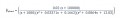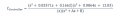# Controller for plant transfer function.

#### Saviour Muscat

Joined Sep 19, 2014
180
Hello,
Hope this thread finds you in good health,

Currently I'm doing a task given for homework and I'm little bit stuck. The task is to design a controller to minimize the effect of the two pairs of dominant poles by using pole-zero cancellation technique. The plant transfer function(G) is as follows:
(0.02 (s+1e05))
G=-------------------------------------------------------------------
((s+1000) (s^2 + 0.03371s + 0.1662) (s^2 + 0.0864s + 12.03))

I did an attempt for the controller(C) see below, I placed two pairs of complex zeros at the numerator(C) to cancel out the two pairs of complex poles found in the denominator of (G), further I placed at the denominator at (C): an integrator(to cancel out the steady state error) and complex pole to achieve the design specs( 2 seconds settling time and 5% overshoot).

C=(s^2 + 0.03371s + 0.1662) (s^2 + 0.0864s + 12.03)
---------------------------------------------------------
(s)(s^2+As+B)

My difficulty is it permissible to apply controller (C) with (G)? Or suggest any modifications of controller (C) that is compatible with (G), please.

(s^2 + 0.03371s + 0.1662) (s^2 + 0.0864s + 12.03) (0.02 (s+1e05))
--------------------------------------------------------- ----- --------------------------------------------------------------
(s)(s^2+As+B) ((s+1000) (s^2 + 0.03371s + 0.1662) (s^2 + 0.0864s + 12.03))

Thank you
SM